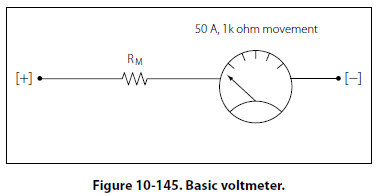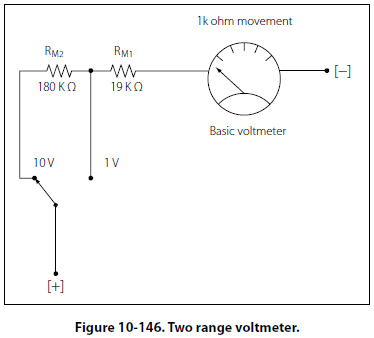Precautions The precautions to observe when using an ammeter are summarized as follows: Always connect an ammeter in series with the element through which the current flow is to be measured. Never connect an ammeter across a source of voltage, such as a battery or generator. Remember that the resistance of an ammeter, particularly on the higher ranges, is extremely low and that any voltage, even a volt or so, can cause very high current to flow through the meter, causing damage to it. Use a range large enough to keep the deflection less than full scale. Before measuring a current, form some idea of its magnitude. Then switch to a large enough scale or start with the highest range and work down until the appropriate scale is reached. The most accurate readings are obtained at approximately half-scale deflection. Many milliammeters have been ruined by attempts to measure amperes. Therefore, be sure to read the lettering either on the dial or on the switch positions and choose proper scale before connecting the meter in the circuit. Observe proper polarity in connecting the meter in the circuit. Current must flow through the coil in a definite direction in order to move the indicator needle up scale. Current reversal because of incorrect connection in the circuit results in a reversed meter deflection and frequently causes bending of the meter needle. Avoid improper meter connections by observing the polarity markings on the meter. The Voltmeter The voltmeter uses the same type of meter movement as the ammeter but employs a different circuit external to the meter movement. As shown before, the voltage drop across the meter coil is a function of current and the coil resistance. In another example, 50µA × 1000O = 50mV. In order for the meter to be used to measure voltages greater than 50mV, there must be added a series resistance to drop any excess voltage greater than that which the meter movement requires for a full scale deflection. The case of the voltmeter, this resistance is called multiplier resistance and will be designated as RM. Figure 10- 145 illustrates a basic voltmeter. This voltmeter only has one multiplier resistor for use in one range. In this example, the full scale reading will be 1 volt. RM is determined in the follow way: The meter movement drops 50mV at a full scale deflection of 50µA. The multiplying resistor RM must drop the remaining voltage of 1V - 50mV = 950 mV. Since RM is in series with the movement, it also carries 50µA at full scale. RM =950 mV/50µA = 19k O Therefore, for 1 volt full scale deflection, the total resistance of the voltmeter is 20 k O. That is, the multiplier resistance and the coil resistance. Voltmeter Sensitivity Voltmeter sensitivity is defined in terms of resistance per volt (O/V). The meter used in the previous example has a sensitivity of 20 k O and a full scale deflection of 1 volt. Multiple Range Voltmeters The simplified voltmeter in Figure 10-145 has only one range (1 volt), which means that it can measure voltages from 0 volts to 1 volt. In order for the meter to be more useful, additional multiplier resistors must be used. One resistor must be used for each desired range.For a 50µA movement, the total resistance required is 20 k O for each volt of full scale reading. In other words, the sensitivity for a 50µA movement is always 20 k O regardless of the selected range. The full-scale meter current is 50µA at any range selection. To find the total meter resistance, multiply the sensitivity by the full scale voltage for that particular range. For example for a 10 volt range, RT = (20k O/V)/(10 V) = 200k O. The total resistance for the 1 volt range is 20k O, so RM for a 10 V range will be 200k O - 20k O = 180k O. This two-range voltmeter is illustrated in Figure 10-146.©AvStop Online Magazine                                                                                                                                                      Contact Us              Return To Books